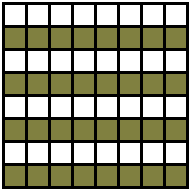# Tiling a Rectangle with L-tetrominoes

Find a necessary and sufficient condition that an a×b rectangle can be exactly covered (completely, and without overlaps) with L-tetrominoes.

The problem has been posted by G. W. Golomb in 1962 and the solution below is due to D. A. Klarner, Humboldt State College, Arcata, California (The American Mathematical Monthly, Vol. 70, No. 7 (Aug. - Sep., 1963), pp. 760-761)### Solutions

Find a necessary and sufficient condition that an a×b rectangle can be exactly covered (completely, and without overlaps) with L-tetrominoes.

### Solution

Since 4 divides ab, a may be taken even. Let a/2 alternate rows of b squares each be colored black in the rectangle.Then every L-tetromino in the covering must cover three squares of one color and one square of the other. If m L-tetrominoes cover three black squares and n L-tetrominoes cover three white squares, then 3m + n = ab/2 = 3n + m; hence m = n. This means that the covering must use an even number of L-tetrominoes, and hence that 8 divides ab. Except for the 1×8k rectangle, every rectangle of area 8k can be partitioned into exhaustive, disjoint rectangles of dimensions 2×4 and/or 3×8, but both the 2×4 and 3×8 rectangle can be packed with L-tetrominoes in an obvious way. Hence, the necessary and sufficient conditions are that a and b be greater than 1 and ab = 8k.### Related materialRead more...

• Covering A Chessboard With Domino
• Dominoes on a Chessboard
• Tiling a Chessboard with Dominoes
• Vertical and Horizontal Dominoes on a Chessboard
• Straight Tromino on a Chessboard
• Golomb's inductive proof of a tromino theorem
• Tromino Puzzle: Interactive Illustration of Golomb's Theorem
• Tromino as a Rep-tile
• Tiling Rectangles with L-Trominoes
• Squares and Straight Tetrominoes
• Covering a Chessboard with a Hole with L-Trominoes
• Tromino Puzzle: Deficient Squares
• Tiling a Square with Tetrominoes Fault-Free
• Tiling a Square with T-, L-, and a Square Tetrominoes
• Tiling a 12x12 Square with Straight Trominoes
• Bicubal Domino
•